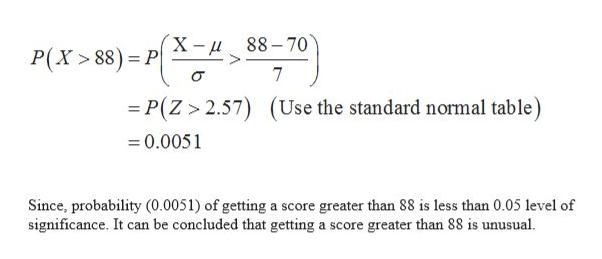# Scores on a statistics final in a large class were normally distributed with a mean of 70 and a standard deviation of 7. Find the following probabilities, round to the fourth.a) What is the probability one randomly chosen score is greater than 88Would it be unusual for this to happen?Select an answer not unusual unusual  b) What proportion of scores were below a 45? Would it be unusual for this to happen?Select an answer unusual not unusual

Question
112 views

Scores on a statistics final in a large class were normally distributed with a mean of 70 and a standard deviation of 7. Find the following probabilities, round to the fourth.
a) What is the probability one randomly chosen score is greater than 88

Would it be unusual for this to happen?
Select an answer not unusual unusual
b) What proportion of scores were below a 45?
Would it be unusual for this to happen?
Select an answer unusual not unusual

check_circle

Step 1

a) According to the provided information, it is given µ = 70 and σ = 7

The probability that one...help_outlineImage Transcriptionclose88-70 X- P(X> 88) P 7 P(Z> 2.57) (Use the standard normal table) =0.0051 Since, probability (0.0051) of getting a score greater than 88 is less than 0.05 level of significance. It can be concluded that getting a score greater than 88 is unusual. fullscreen

### Want to see the full answer?

See Solution

#### Want to see this answer and more?

Solutions are written by subject experts who are available 24/7. Questions are typically answered within 1 hour.*

See Solution
*Response times may vary by subject and question.
Tagged in

### Statistics